Скачать презентацию EE 515 IS 523 Think Like an Adversary Lecture

02be7607cfbe4f800e5fb1bdbe6c5acb.ppt

• Количество слайдов: 37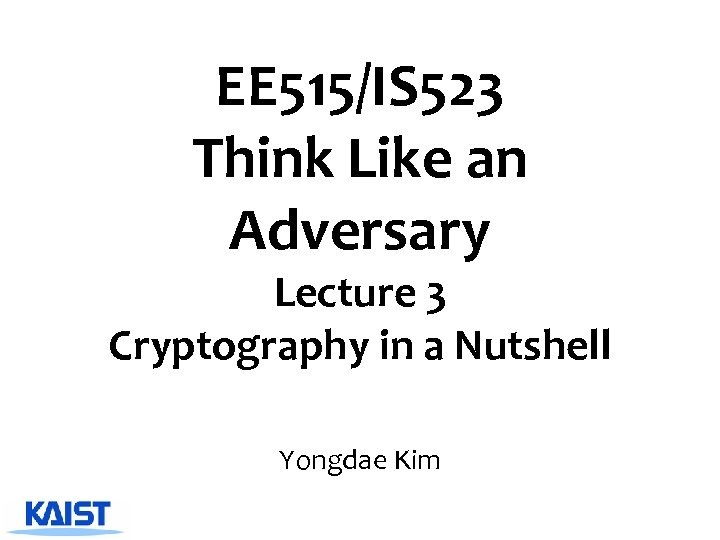EE 515/IS 523 Think Like an Adversary Lecture 3 Cryptography in a Nutshell Yongdae Kim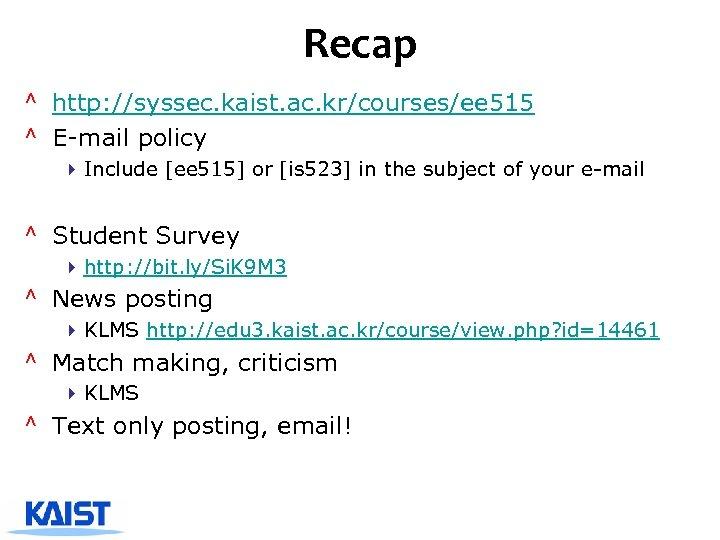Recap ^ http: //syssec. kaist. ac. kr/courses/ee 515 ^ E-mail policy 4 Include [ee 515] or [is 523] in the subject of your e-mail ^ Student Survey 4 http: //bit. ly/Si. K 9 M 3 ^ News posting 4 KLMS http: //edu 3. kaist. ac. kr/course/view. php? id=14461 ^ Match making, criticism 4 KLMS ^ Text only posting, email!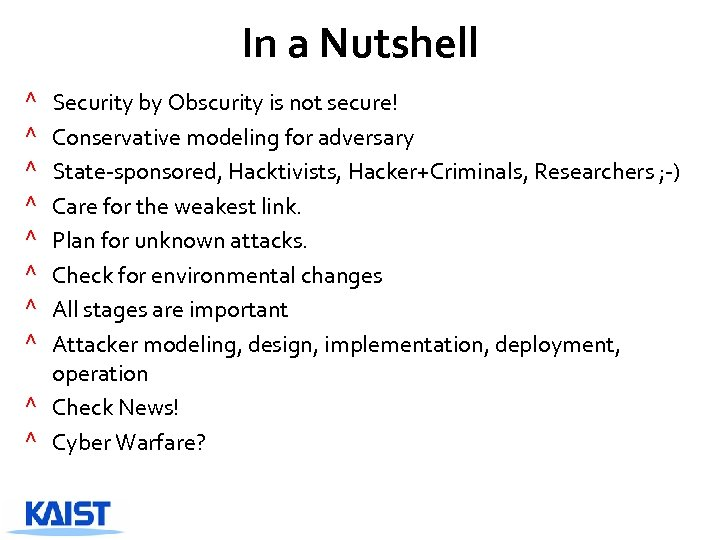In a Nutshell Security by Obscurity is not secure! Conservative modeling for adversary State-sponsored, Hacktivists, Hacker+Criminals, Researchers ; -) Care for the weakest link. Plan for unknown attacks. Check for environmental changes All stages are important Attacker modeling, design, implementation, deployment, operation ^ Check News! ^ Cyber Warfare? ^ ^ ^ ^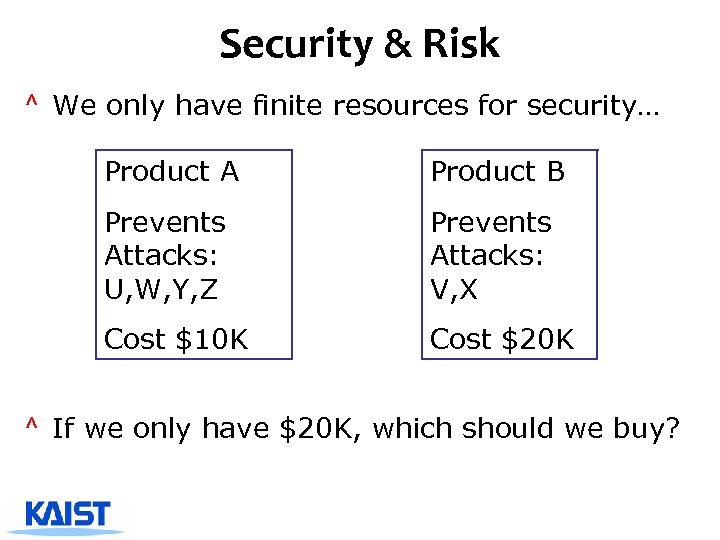Security & Risk ^ We only have finite resources for security… Product A Product B Prevents Attacks: U, W, Y, Z Prevents Attacks: V, X Cost \$10 K Cost \$20 K ^ If we only have \$20 K, which should we buy?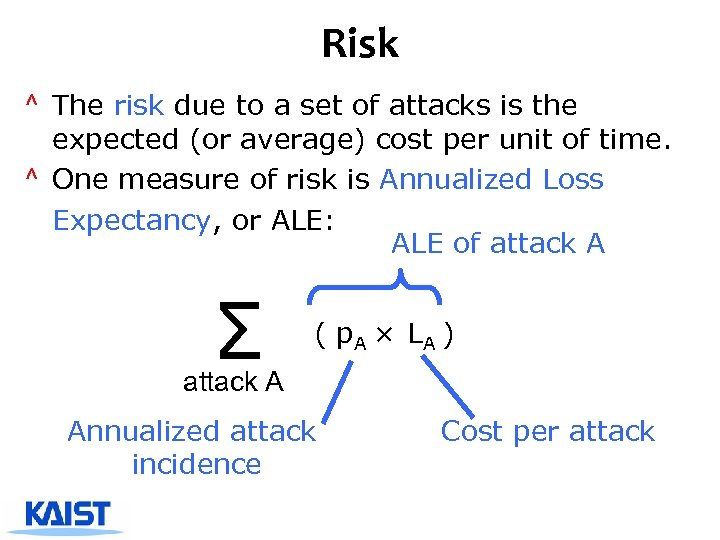Risk ^ The risk due to a set of attacks is the expected (or average) cost per unit of time. ^ One measure of risk is Annualized Loss Expectancy, or ALE: ALE of attack A Σ ( p. A × LA ) attack A Annualized attack incidence Cost per attack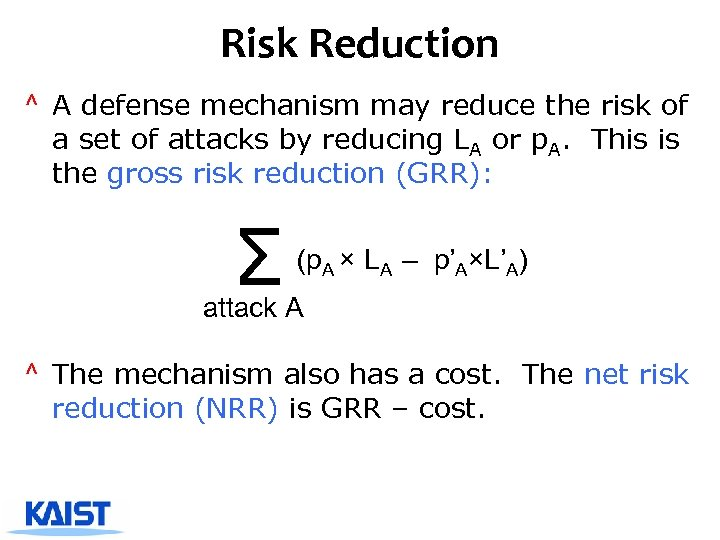Risk Reduction ^ A defense mechanism may reduce the risk of a set of attacks by reducing LA or p. A. This is the gross risk reduction (GRR): Σ (p. A × LA – p’A×L’A) attack A ^ The mechanism also has a cost. The net risk reduction (NRR) is GRR – cost.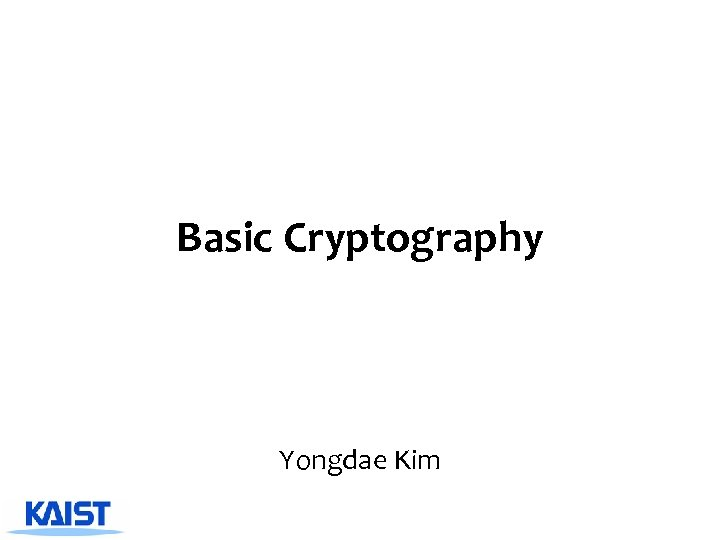Basic Cryptography Yongdae Kim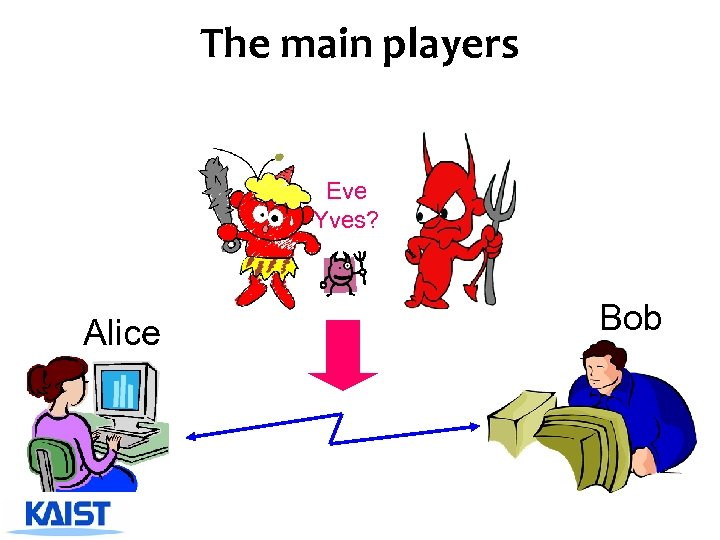The main players Eve Yves? Alice Bob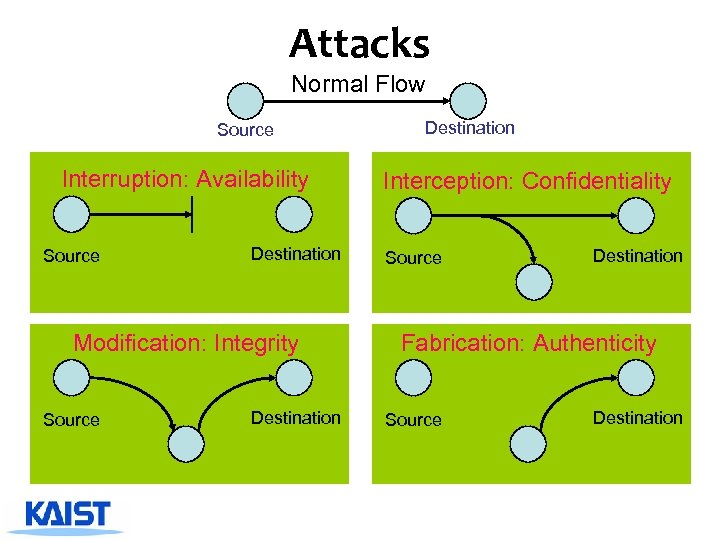Attacks Normal Flow Source Interruption: Availability Source Destination Modification: Integrity Source Destination Interception: Confidentiality Source Destination Fabrication: Authenticity Source Destination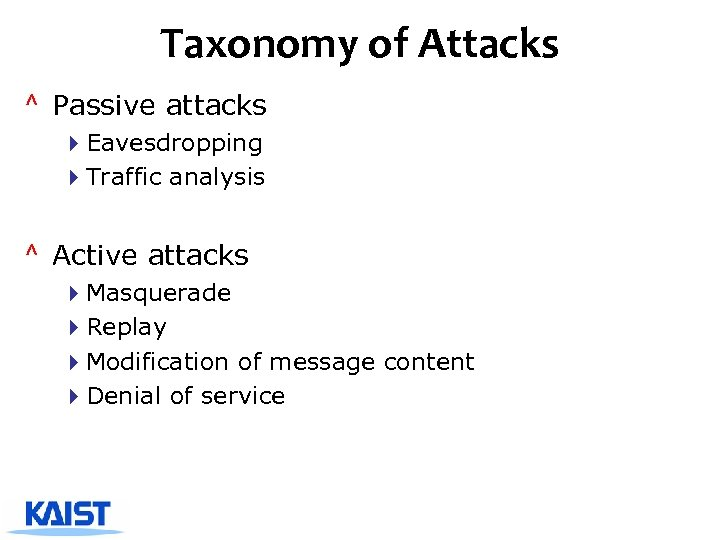Taxonomy of Attacks ^ Passive attacks 4 Eavesdropping 4 Traffic analysis ^ Active attacks 4 Masquerade 4 Replay 4 Modification of message content 4 Denial of service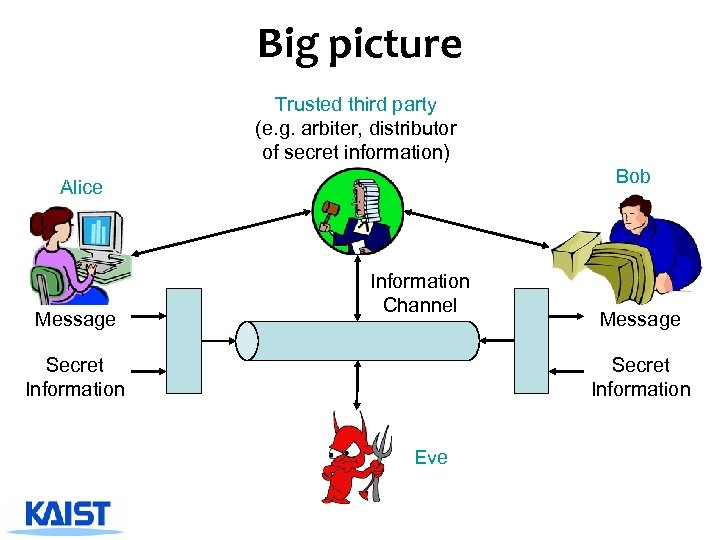Big picture Trusted third party (e. g. arbiter, distributor of secret information) Bob Alice Message Information Channel Secret Information Message Secret Information Eve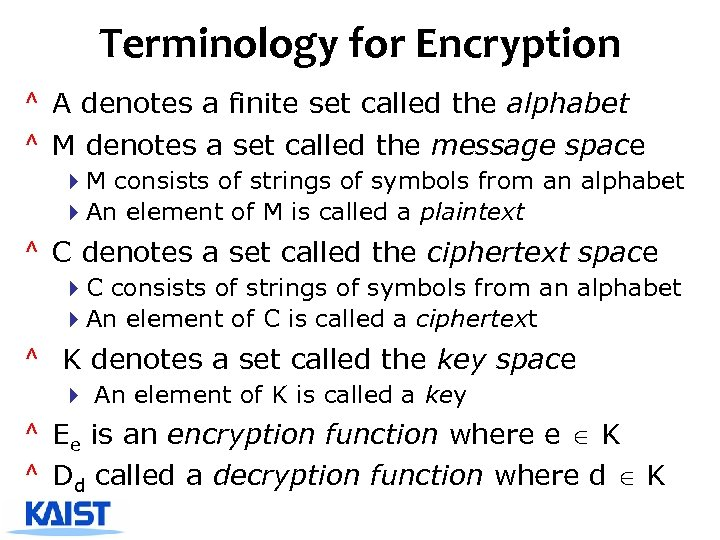Terminology for Encryption ^ A denotes a finite set called the alphabet ^ M denotes a set called the message space 4 M consists of strings of symbols from an alphabet 4 An element of M is called a plaintext ^ C denotes a set called the ciphertext space 4 C consists of strings of symbols from an alphabet 4 An element of C is called a ciphertext ^ K denotes a set called the key space 4 An element of K is called a key ^ Ee is an encryption function where e K ^ Dd called a decryption function where d K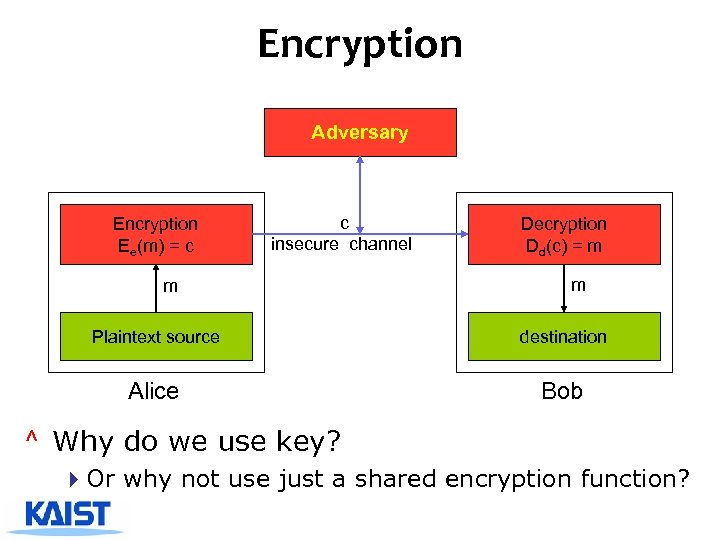Encryption Adversary Encryption Ee(m) = c c insecure channel m Decryption Dd(c) = m m Plaintext source destination Alice Bob ^ Why do we use key? 4 Or why not use just a shared encryption function?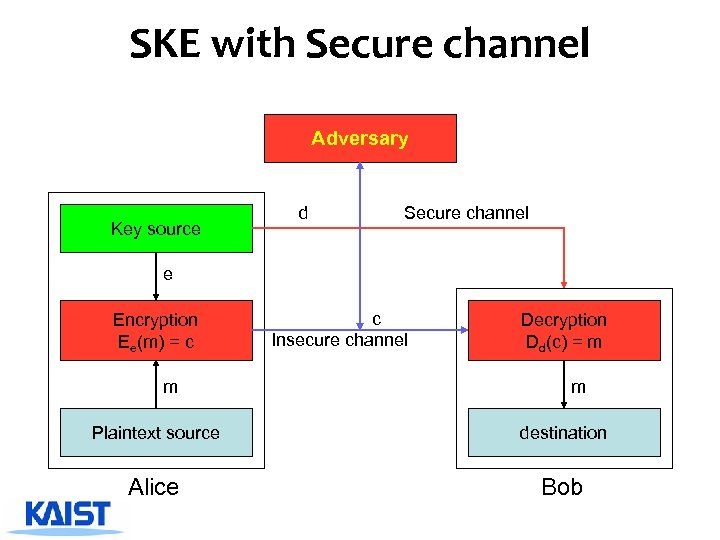SKE with Secure channel Adversary Key source d Secure channel e Encryption Ee(m) = c m c Insecure channel Decryption Dd(c) = m m Plaintext source destination Alice Bob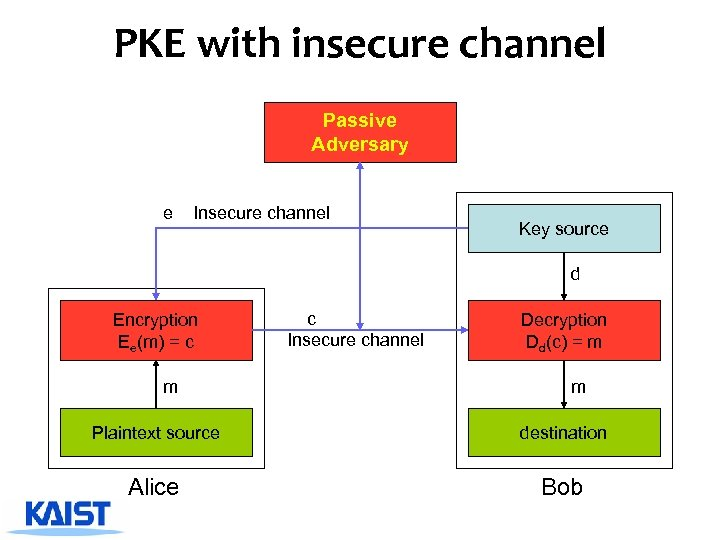PKE with insecure channel Passive Adversary e Insecure channel Key source d Encryption Ee(m) = c m c Insecure channel Decryption Dd(c) = m m Plaintext source destination Alice Bob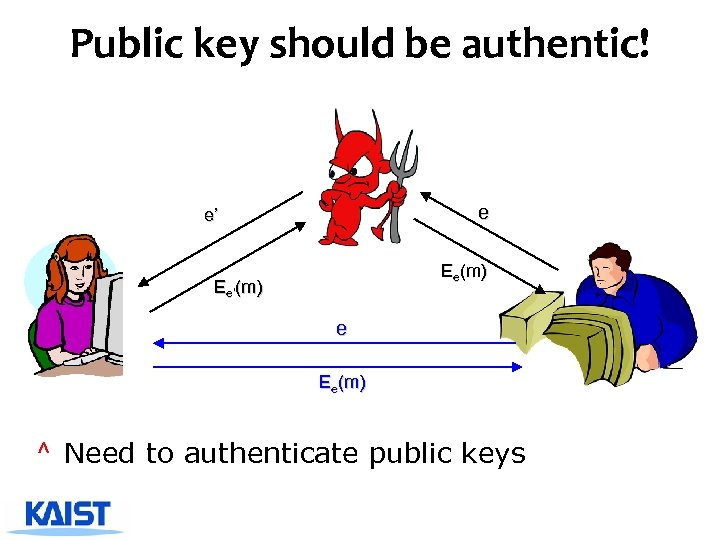Public key should be authentic! e e’ Ee(m) Ee’(m) e Ee(m) ^ Need to authenticate public keys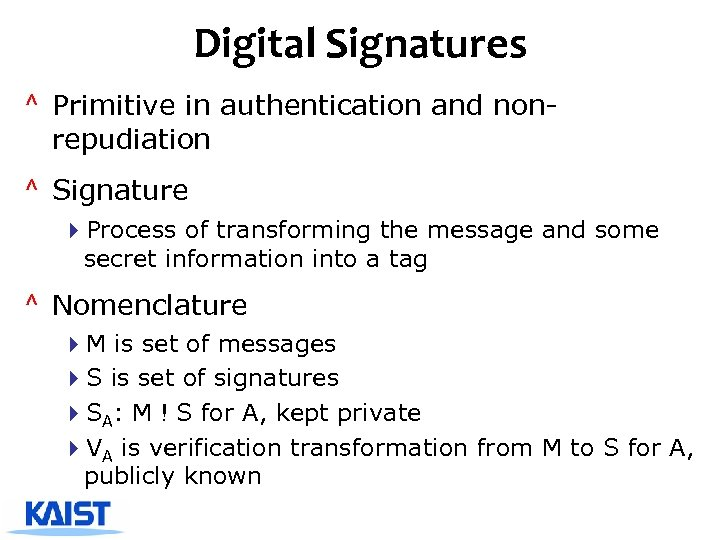Digital Signatures ^ Primitive in authentication and nonrepudiation ^ Signature 4 Process of transforming the message and some secret information into a tag ^ Nomenclature 4 M is set of messages 4 S is set of signatures 4 SA: M ! S for A, kept private 4 VA is verification transformation from M to S for A, publicly known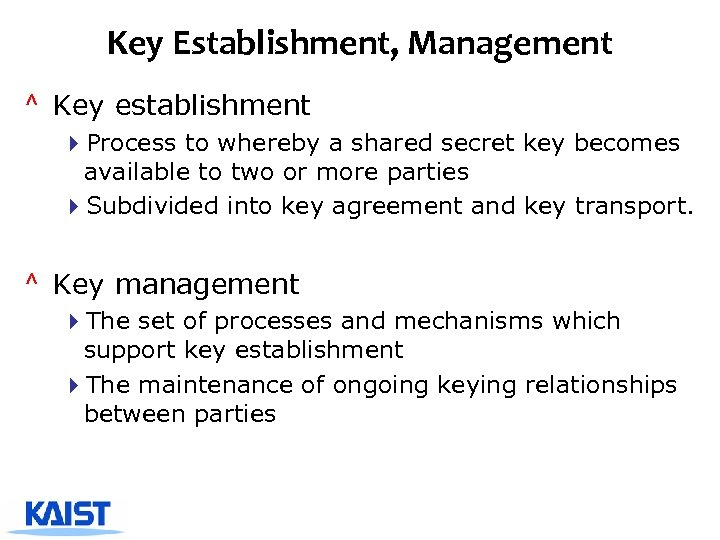Key Establishment, Management ^ Key establishment 4 Process to whereby a shared secret key becomes available to two or more parties 4 Subdivided into key agreement and key transport. ^ Key management 4 The set of processes and mechanisms which support key establishment 4 The maintenance of ongoing keying relationships between parties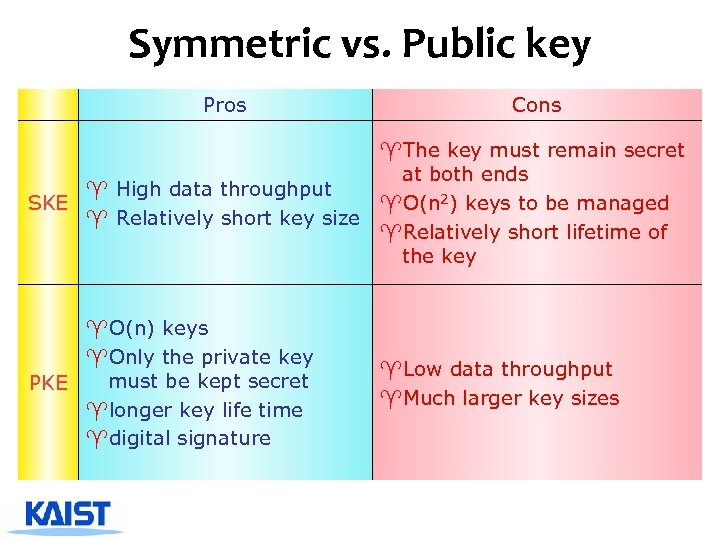Symmetric vs. Public key Pros Cons ^The key must remain secret at both ends ^ High data throughput SKE ^O(n 2) keys to be managed ^ Relatively short key size ^Relatively short lifetime of the key ^O(n) keys ^Only the private key must be kept secret PKE ^longer key life time ^digital signature ^Low data throughput ^Much larger key sizes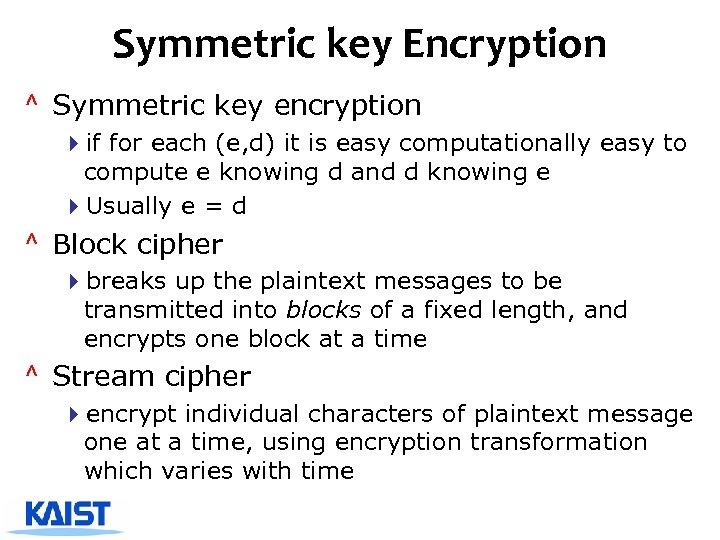Symmetric key Encryption ^ Symmetric key encryption 4 if for each (e, d) it is easy computationally easy to compute e knowing d and d knowing e 4 Usually e = d ^ Block cipher 4 breaks up the plaintext messages to be transmitted into blocks of a fixed length, and encrypts one block at a time ^ Stream cipher 4 encrypt individual characters of plaintext message one at a time, using encryption transformation which varies with time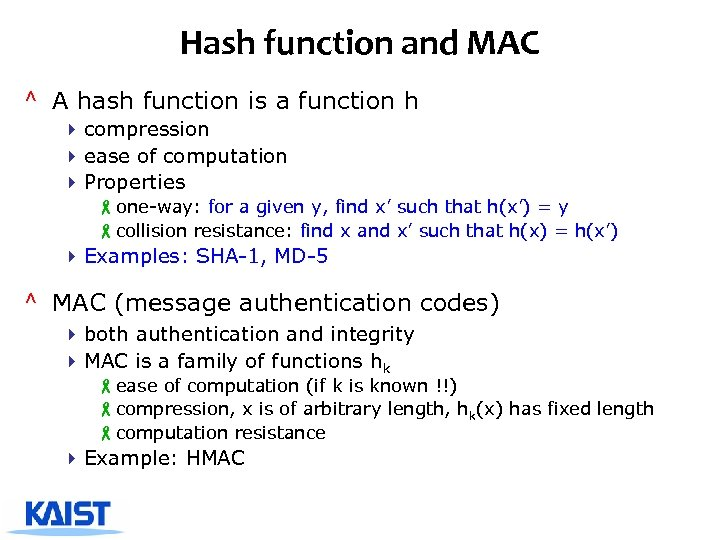Hash function and MAC ^ A hash function is a function h 4 compression 4 ease of computation 4 Properties -one-way: for a given y, find x’ such that h(x’) = y -collision resistance: find x and x’ such that h(x) = h(x’) 4 Examples: SHA-1, MD-5 ^ MAC (message authentication codes) 4 both authentication and integrity 4 MAC is a family of functions hk -ease of computation (if k is known !!) -compression, x is of arbitrary length, hk(x) has fixed length -computation resistance 4 Example: HMAC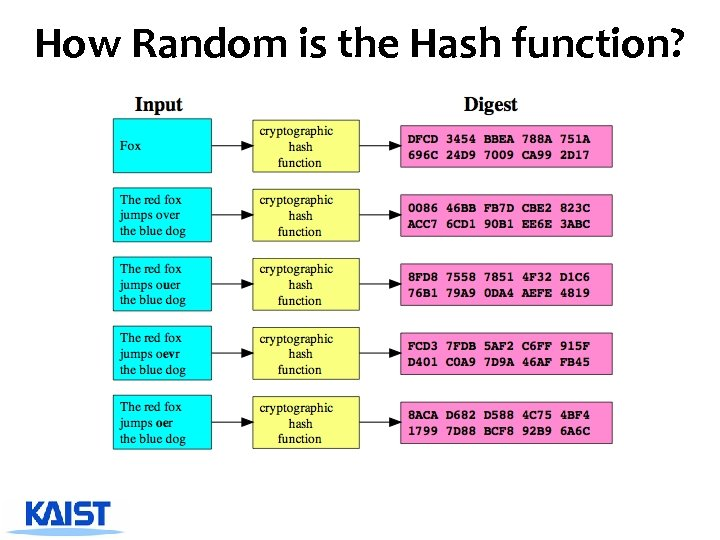How Random is the Hash function?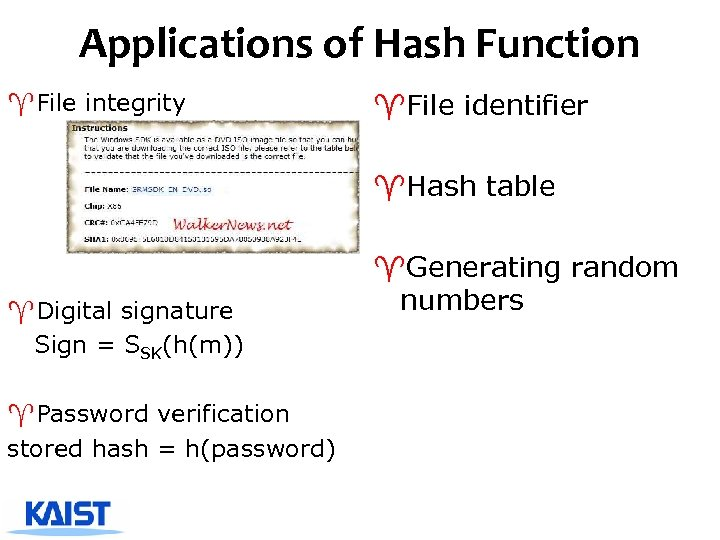Applications of Hash Function ^File integrity ^File identifier ^Hash table ^Digital signature Sign = SSK(h(m)) ^Password verification stored hash = h(password) ^Generating random numbers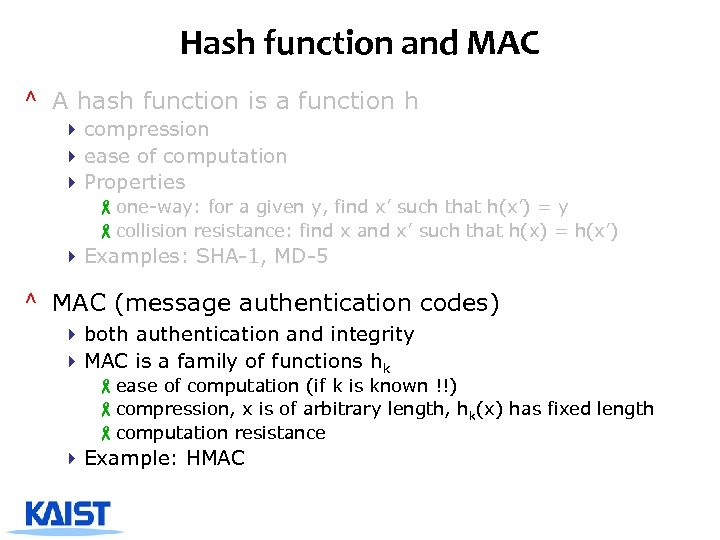Hash function and MAC ^ A hash function is a function h 4 compression 4 ease of computation 4 Properties -one-way: for a given y, find x’ such that h(x’) = y -collision resistance: find x and x’ such that h(x) = h(x’) 4 Examples: SHA-1, MD-5 ^ MAC (message authentication codes) 4 both authentication and integrity 4 MAC is a family of functions hk -ease of computation (if k is known !!) -compression, x is of arbitrary length, hk(x) has fixed length -computation resistance 4 Example: HMAC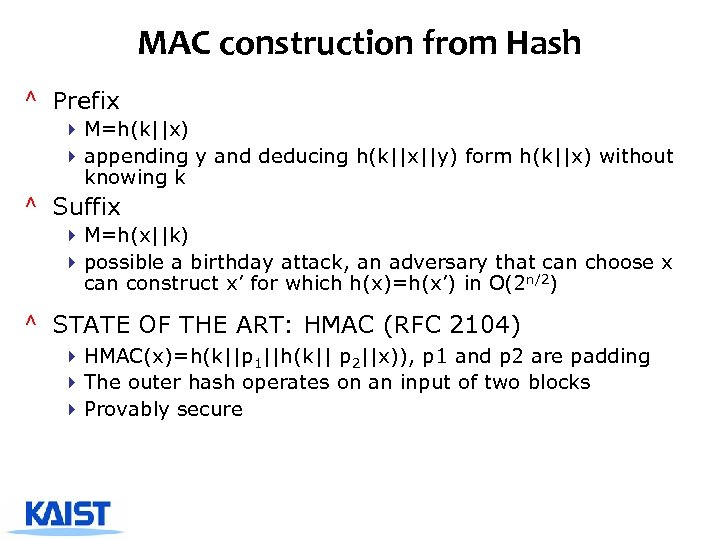MAC construction from Hash ^ Prefix 4 M=h(k||x) 4 appending y and deducing h(k||x||y) form h(k||x) without knowing k ^ Suffix 4 M=h(x||k) 4 possible a birthday attack, an adversary that can choose x can construct x’ for which h(x)=h(x’) in O(2 n/2) ^ STATE OF THE ART: HMAC (RFC 2104) 4 HMAC(x)=h(k||p 1||h(k|| p 2||x)), p 1 and p 2 are padding 4 The outer hash operates on an input of two blocks 4 Provably secure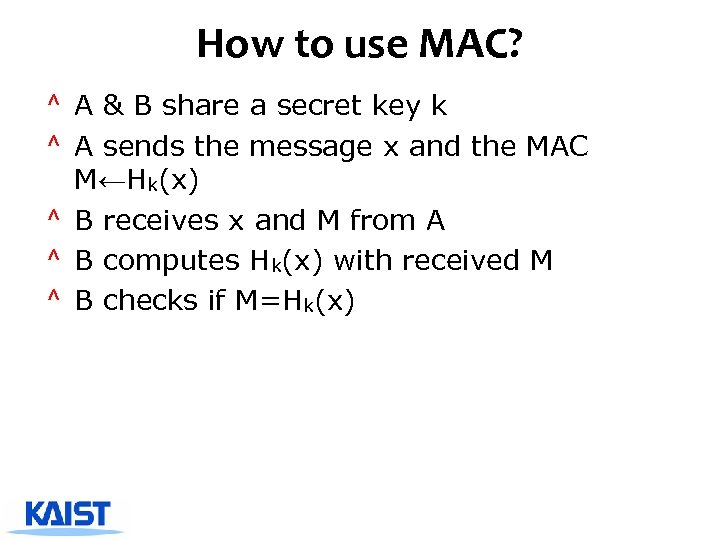How to use MAC? ^ A & B share a secret key k ^ A sends the message x and the MAC M←Hk(x) ^ B receives x and M from A ^ B computes Hk(x) with received M ^ B checks if M=Hk(x)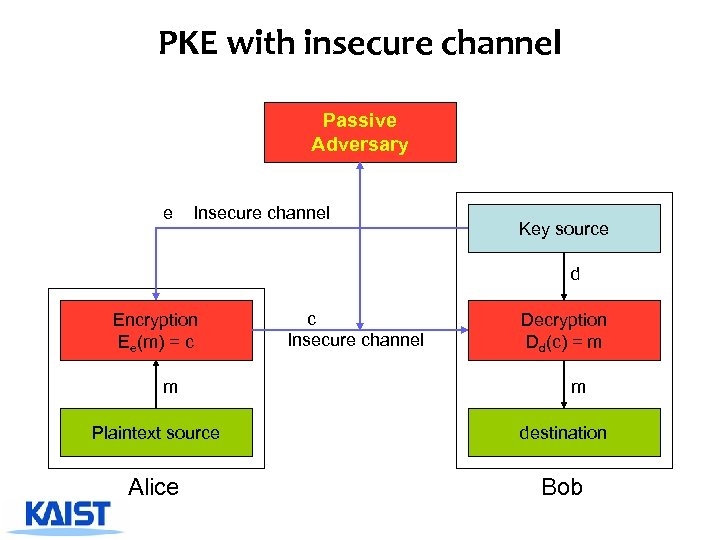PKE with insecure channel Passive Adversary e Insecure channel Key source d Encryption Ee(m) = c m c Insecure channel Decryption Dd(c) = m m Plaintext source destination Alice Bob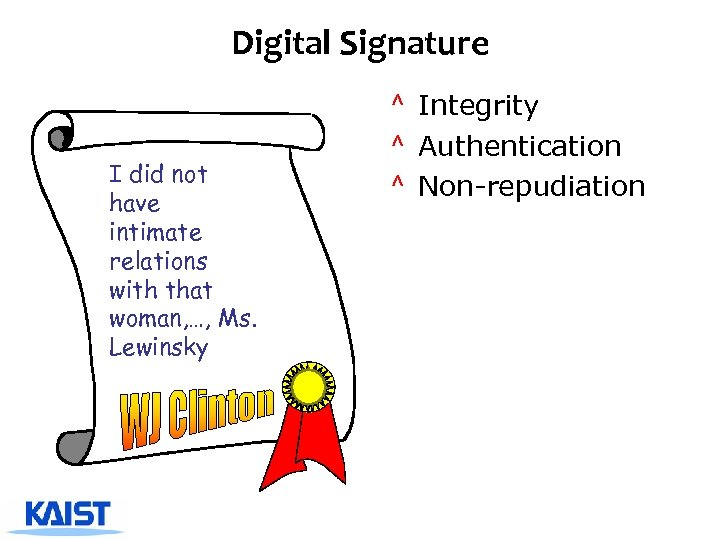Digital Signature I did not have intimate relations with that woman, …, Ms. Lewinsky ^ Integrity ^ Authentication ^ Non-repudiation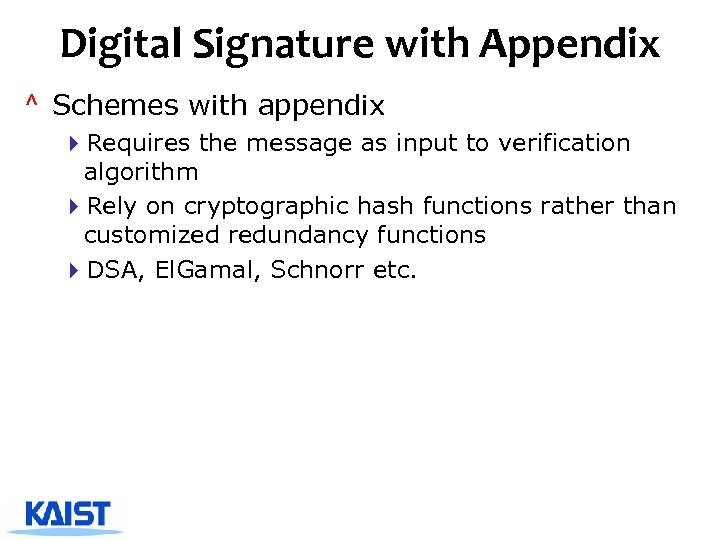Digital Signature with Appendix ^ Schemes with appendix 4 Requires the message as input to verification algorithm 4 Rely on cryptographic hash functions rather than customized redundancy functions 4 DSA, El. Gamal, Schnorr etc.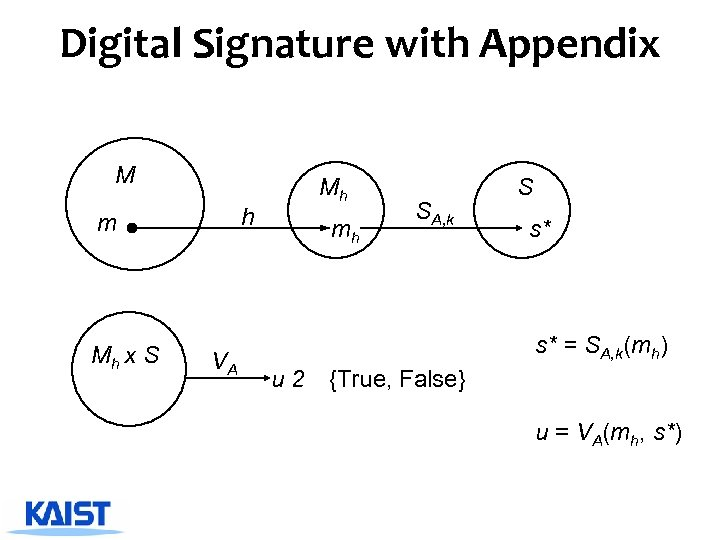Digital Signature with Appendix M h m Mh x S VA Mh mh SA, k S s* s* = SA, k(mh) u 2 {True, False} u = VA(mh, s*)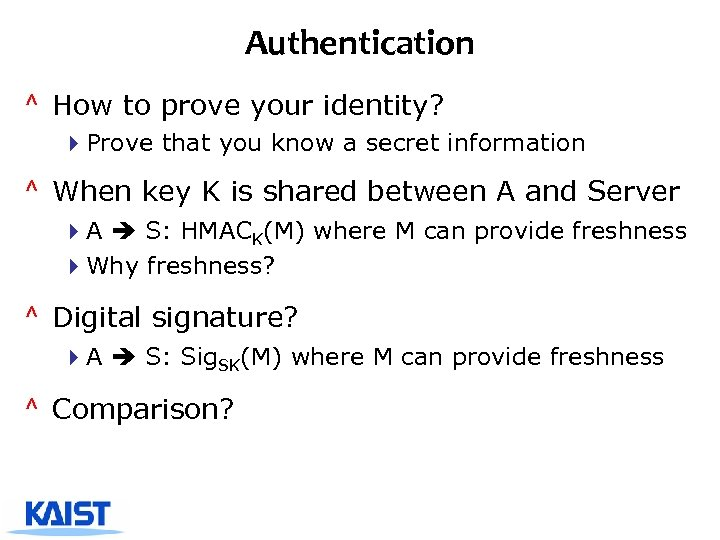Authentication ^ How to prove your identity? 4 Prove that you know a secret information ^ When key K is shared between A and Server 4 A S: HMACK(M) where M can provide freshness 4 Why freshness? ^ Digital signature? 4 A S: Sig. SK(M) where M can provide freshness ^ Comparison?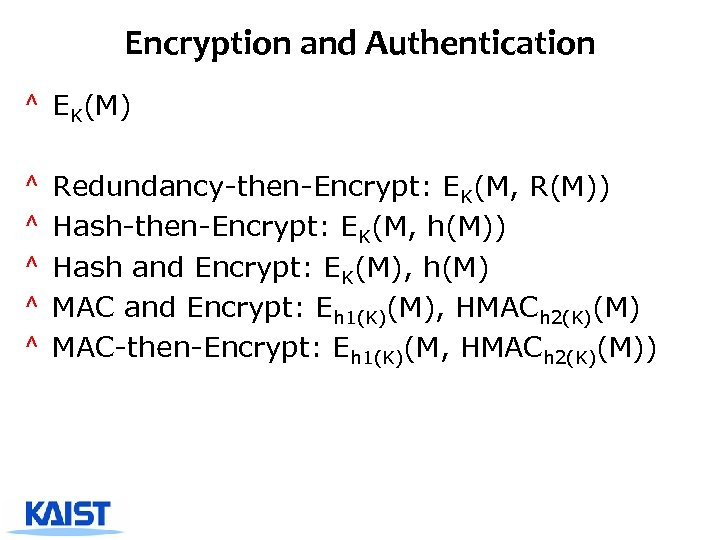Encryption and Authentication ^ EK(M) ^ ^ ^ Redundancy-then-Encrypt: EK(M, R(M)) Hash-then-Encrypt: EK(M, h(M)) Hash and Encrypt: EK(M), h(M) MAC and Encrypt: Eh 1(K)(M), HMACh 2(K)(M) MAC-then-Encrypt: Eh 1(K)(M, HMACh 2(K)(M))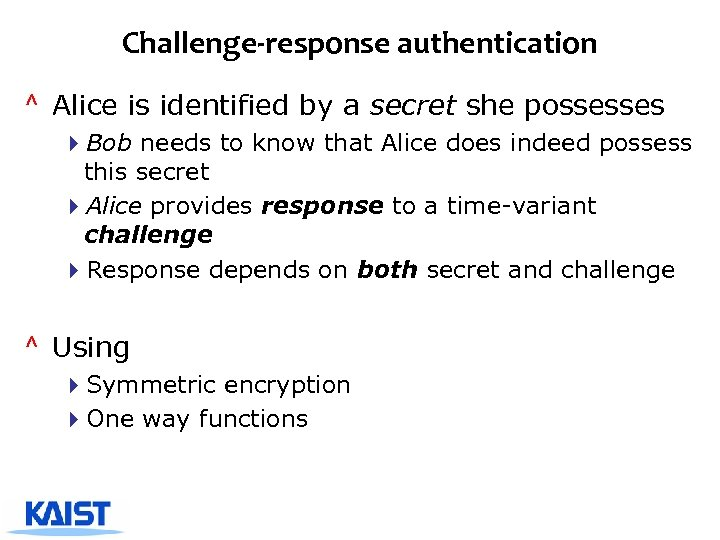Challenge-response authentication ^ Alice is identified by a secret she possesses 4 Bob needs to know that Alice does indeed possess this secret 4 Alice provides response to a time-variant challenge 4 Response depends on both secret and challenge ^ Using 4 Symmetric encryption 4 One way functions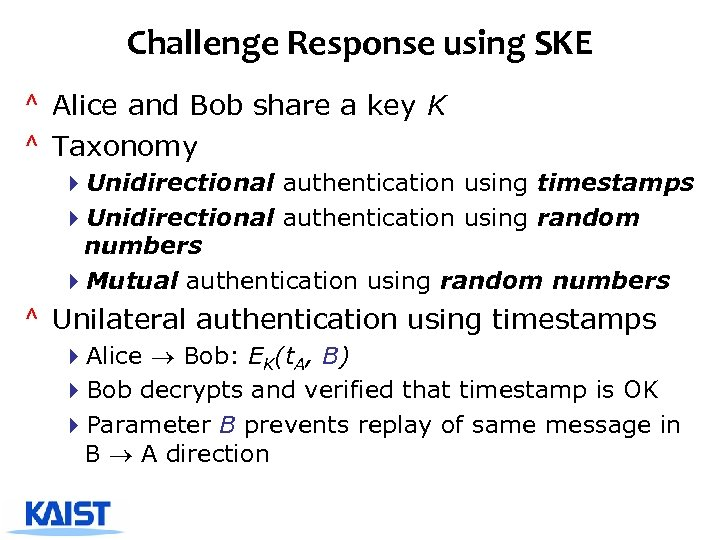Challenge Response using SKE ^ Alice and Bob share a key K ^ Taxonomy 4 Unidirectional authentication using timestamps 4 Unidirectional authentication using random numbers 4 Mutual authentication using random numbers ^ Unilateral authentication using timestamps 4 Alice Bob: EK(t. A, B) 4 Bob decrypts and verified that timestamp is OK 4 Parameter B prevents replay of same message in B A direction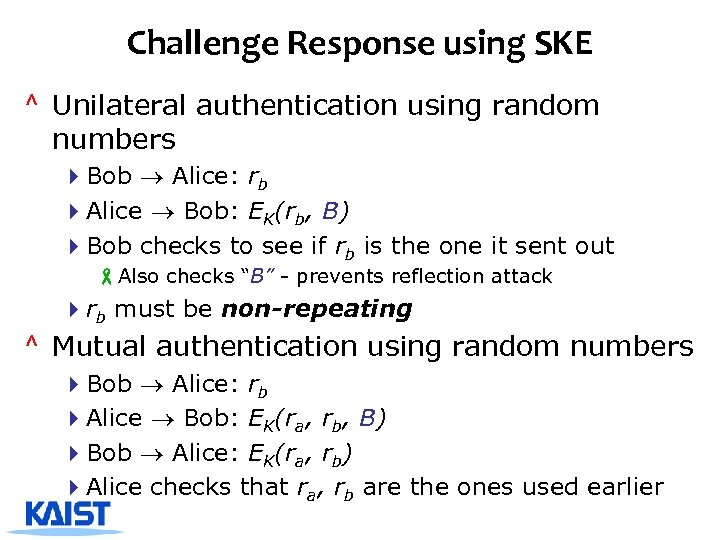Challenge Response using SKE ^ Unilateral authentication using random numbers 4 Bob Alice: rb 4 Alice Bob: EK(rb, B) 4 Bob checks to see if rb is the one it sent out -Also checks “B” - prevents reflection attack 4 rb must be non-repeating ^ Mutual authentication using random numbers 4 Bob Alice: rb 4 Alice Bob: EK(ra, rb, B) 4 Bob Alice: EK(ra, rb) 4 Alice checks that ra, rb are the ones used earlier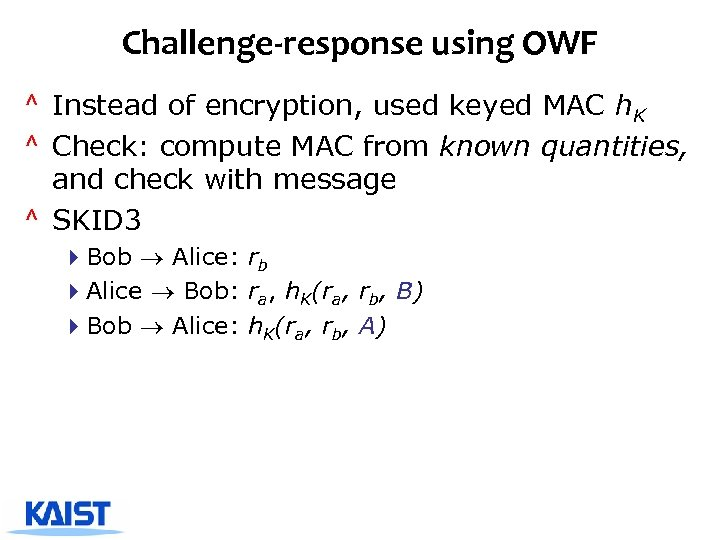Challenge-response using OWF ^ Instead of encryption, used keyed MAC h. K ^ Check: compute MAC from known quantities, and check with message ^ SKID 3 4 Bob Alice: rb 4 Alice Bob: ra, h. K(ra, rb, B) 4 Bob Alice: h. K(ra, rb, A)Key Establishment, Management ^ Key establishment 4 Process to whereby a shared secret key becomes available to two or more parties 4 Subdivided into key agreement and key transport. ^ Key management 4 The set of processes and mechanisms which support key establishment 4 The maintenance of ongoing keying relationships between parties